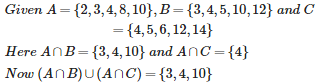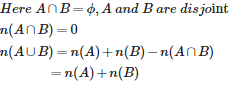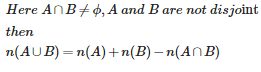# Maths Sets - Online Test

Q1. Two sets A and B are said to be disjoint iff
Explaination / Solution:

Two sets are called disjoint if and only if these two set have no common element i.e A∩B=∅

Q2. Which of the following is a null set ?
Explaination / Solution:

[i=imaginory root of unity which is a complex no.]

since the solution of x doesnot belongs to real no hence the set is null set

Q3. If A ⊆B , then A∩B is equal to
Explaination / Solution:

A ⊆B means Set A is contained in the set B.So common region is The Set A

Q4. If A ⊆B , then A∪B is equal to
Explaination / Solution:

A ⊆B refers to A set is contained in the Set B.So Set B is bigger.So union the sets will be B

Q5. If A and B are two sets , then A∪(A∩B) is equal to
Explaination / Solution:

LetA={1,2,3,4}andB={1,2,3,4,5,6}HereA∩B={1,2,3,4}NowA∪(A∩B)={1,2,3,4,}=A

Q6. If A and B are two sets , then A∩(A∪B) is equal to
Explaination / Solution:

LetA={1,2,3,4}andB={1,2,3,4,5,6}HereA∪B={1,2,3,4,5,6}NowA∩(A∪B)={1,2,3,4,}=A

Q7. If A = { 2,3,4,8,10 } ,B = { 3,4,5,10,12 } and C = { 4,5,6,12,14 } , then (A∩B)∪(A∩C) then
Explaination / Solution:Q8. If A and B are disjoint Set , then n ( A∪B) is equal to
Explaination / Solution:Q9. If A and B are not disjoint Set , then n (A∪B) is equal to
Explaination / Solution:Q10. The Collection of intelligent students in a class is
Explaination / Solution:

Intelligency Can not measured by numbers i.e the collection is not well defined thats why it can not be called as a Set